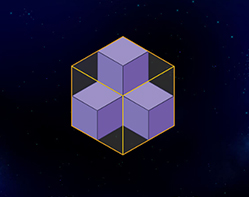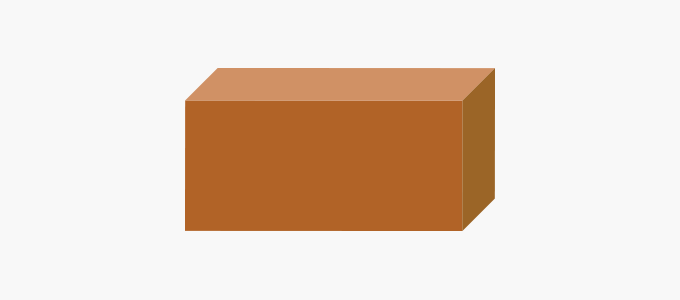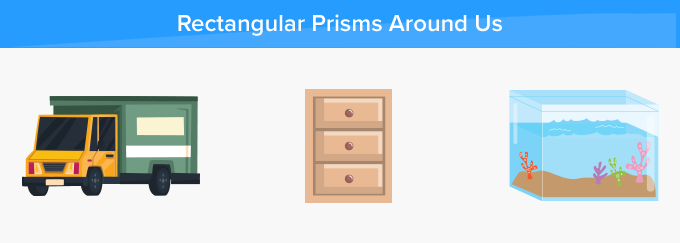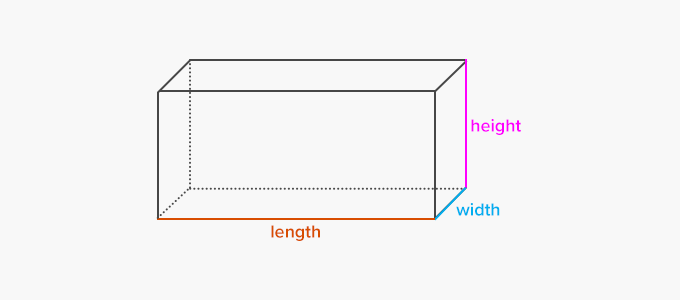# Rectangular Prism - Definition with Examples

The Complete K-5 Math Learning Program Built for Your Child

• 40 Million Kids

Loved by kids and parent worldwide

• 50,000 Schools

Trusted by teachers across schools

• Comprehensive Curriculum

Aligned to Common Core

## Rectangular Prism GamesVolume of Solids

Find the volume of solids by multiplying the area of the base by the height of the solid. Remember, that volume like area can be added.

Covers Common Core Curriculum 5.MD.5.c

## What is a Rectangular Prism?

In geometry, a rectangular prism can be defined as a 3-dimensional solid shape which has six faces that are rectangles.

A rectangular prism is also a cuboid.We can find the shape of a rectangular prism in a truck, a chest of drawers and in an aquarium, around us.## Properties of a Rectangular Prism :

• A rectangular prism has 8 vertices, 12 sides and 6 rectangular faces.
• All the opposite faces of a rectangular prism are equal.
• A rectangular prism has a rectangular cross section.

Rectangular prisms can be of two types, namely right rectangular prism and non-right rectangular prisms.

## Surface Area and Volume of a Rectangular Prism:

Let’s consider this rectangular prism with the length, width and height marked.Since all the faces of a rectangular prism are rectangles and opposite faces are equal, the surface area of a rectangular prism can be calculated using the following formula:

 Surface Area = 2 {( width x length ) + ( length x height ) + (width x height )}

Volume of a rectangular prism is simply obtained by multiplying all three dimensions - length, height and width.

 Volume = length x width x height

 Fun Facts Rectangular prisms are the most commonly used prisms in real life, especially in packaging, from cereal boxes to cartons and parcels delivered by mail.

##Let's sing!

I see a rectangular prism in a red brick.
And, sometimes in books, big, fat and thick.
Also, in a suitcase, and the TV remote I click.

##Let's do it!

Ask your child to find and observe things in the shape of rectangular prism such as in a television, a cupboard, a cookie box or a cereal box. Further, you can show and explore how a cereal box is a 3-dimensional rectangular prism.

##Related math vocabulary

Won Numerous Awards & Honors